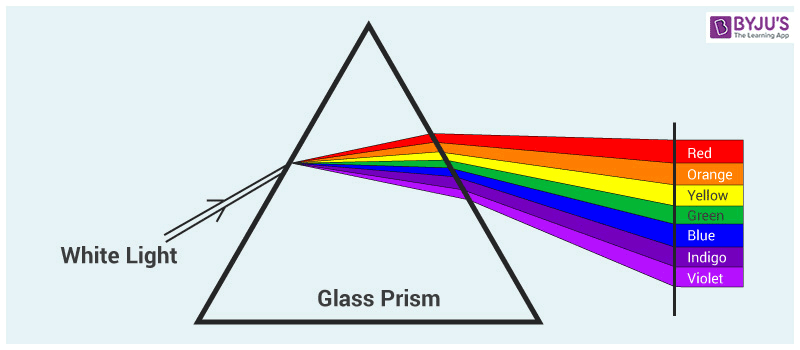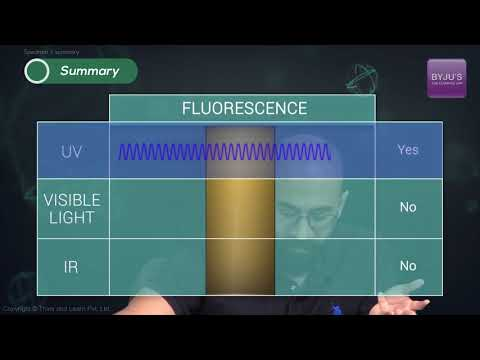# Wavelength of Light

Have you ever thought about the reason how humans are capable of seeing colours? The reason is, colours are nothing but electromagnetic radiations with a different wavelength of light. In the electromagnetic spectrum, the visible region is otherwise called visible light. These colours have different wavelengths too.

## What is Wavelength?

Wavelength is defined as the property of a wave in which the distance between the identical points between the two successive waves are calculated.

It is denoted by the Greek letter lambda (λ). Therefore, the distance between either one crest or trough of one wave and the next wave is known as wavelength.

The wavelength of light is defined as “The distance between the two successive crests or troughs of the light wave”.

## What is Visible Spectrum?

The visible spectrum is nothing but the observable region of the electromagnetic wave which is visible to human eyes. In the electromagnetic spectrum, the visible spectrum ranges from the infrared region to the UV region. The visible light lies in between the infrared and ultraviolet range of wavelengths. The human eye can detect the light spectrum ranging from 400 nanometers (violet) to about 700 nanometers (red). Other electromagnetic radiations are either too small or too large to capture for the human eye and are out of biological limitations.

We can see these waves as the colours of the rainbow where each colour includes a different wavelength. We can also see the sun’s outermost layer – the corona in the visible light.When the visible light travels through a prism, the visible light gets separated into a spectrum of colour. Red colour has the longest wavelength of 700 nm, and violet has the shortest wavelength of 380 nm. These colours arrange themselves according to the wavelength as the spectrum of rainbow colours.

## How is the Wavelength of Light Calculated?

As light has the properties of a wave and a particle, it can be expressed in two equations:

$$\nu =\lambda f$$………………..(1)
$$E =hf$$…………….(2)
Where,

• $$\nu$$ is the velocity of the light.
• $$\lambda$$ is the wavelength of the light.
• $$f$$ is the frequency of the light.
• $$E$$ is the energy of the light wave.
• $$h$$ is the Planck’s Constant i.e. $$6.64\times 10^{-34}joule\cdot second$$

Here, the first equation denotes the wave nature of the light and the second equation denotes the particle nature of the light.

### Wavelength of Visible Light• Wavelength of the Visible Light ranges between 400 nm to 700 nm and here we come to know the wavelength of various colours of the visible spectrum of light.
• The spectrum of visible light has about numerous different colours having different wavelengths.
• The violet colour is said to have the shortest form of wavelength whereas red colour is said to have the longest wavelength.
• It can also be noticed in the below-given figure.The wavelength for various colours of the visible spectrum of light is provided in the table underneath.

 Colour Wavelength Frequency Violet 380-450 nm 668-789 THz Blue 450-495 nm 606-668 THz Green 495-570 nm 526-606 THz Yellow 570-590 nm 508-526 THz Orange 590-620 nm 484-508 THz Red 620-750 nm 400-484 THz

#### Example on Wavelength of Light

Calculate the wavelength of visible light whose frequency is $$6.24\times 10^{14}Hz$$.

Ans: Given, Frequency of light = $$6.24\times 10^{14}Hz$$

We know, velocity of light = $$3\times 10^{8}m/sec$$

As per the formula of wavelength of light,

$$\lambda =\frac{\nu }{f}\\ \\ \Rightarrow \lambda =\frac{3\times 10^{8}}10{6.24\times 10^{14}}\\ \\ \Rightarrow \lambda = 4.80\times 10^{-7}$$

Also, learn the difference between violet and purple.

Since wavelength is inversely proportional to the frequency, we can understand that, longer the wavelength of the light, lower is the frequency. In the same manner, shorter the wavelength, higher will be the frequency of the light.

### What happens to the frequency of the ray of light is traveling from one medium to another?

The frequency of the ray of light remains the same when the ray of light is traveling from one medium to another.

### What are the properties of light waves emitted by the laser?

Following are the properties of the light waves emitted by the laser:

• They have the same phase
• They have the same amplitude
• They have the same frequency

### Why do light waves travel slower in glass than in air?

The light waves travel slower in glass than in air because the density of the glass is greater than the density of the air.

### State if the given statement is true or false: Light cannot travel through vacuum.

The given statement is false because light can travel through the vacuum. When the light wave travels through the vacuum, it is not dependent on the wavelength, frequency, and intensity.

### What happens to the pattern obtained during diffraction if monochromatic light is replaced by the white light?

When the white light replaces monochromatic light, a coloured pattern is observed along with white fringe at the center. Also, the clarity of the band will be lost as there will be overlapping of the coloured bands.

Stay tuned with BYJU’S for more such interesting articles. Also, register to “BYJU’S – The Learning App” for loads of interactive, engaging Physics-related videos and an unlimited academic assist.

Test your knowledge on Wavelength Of Light